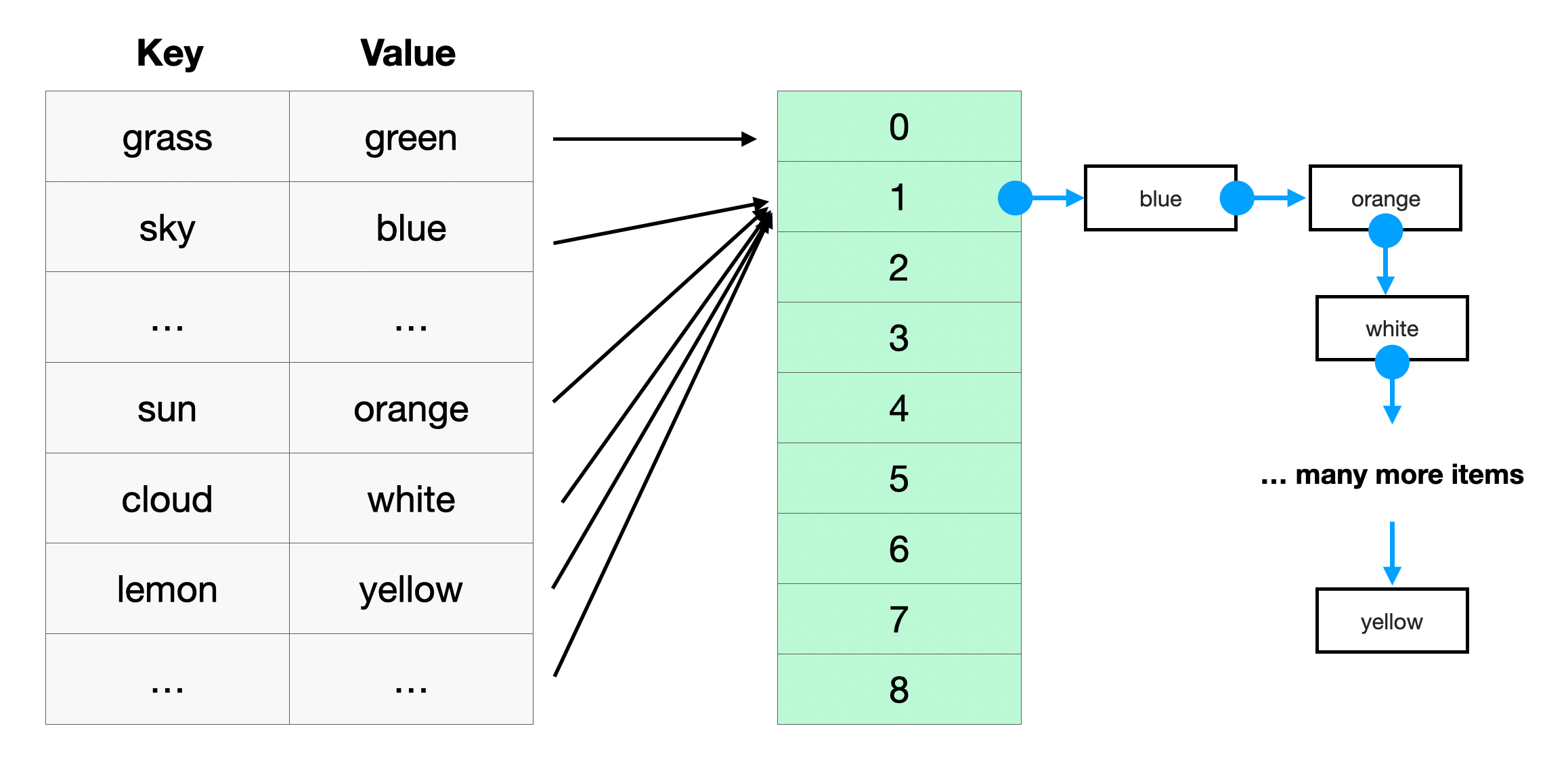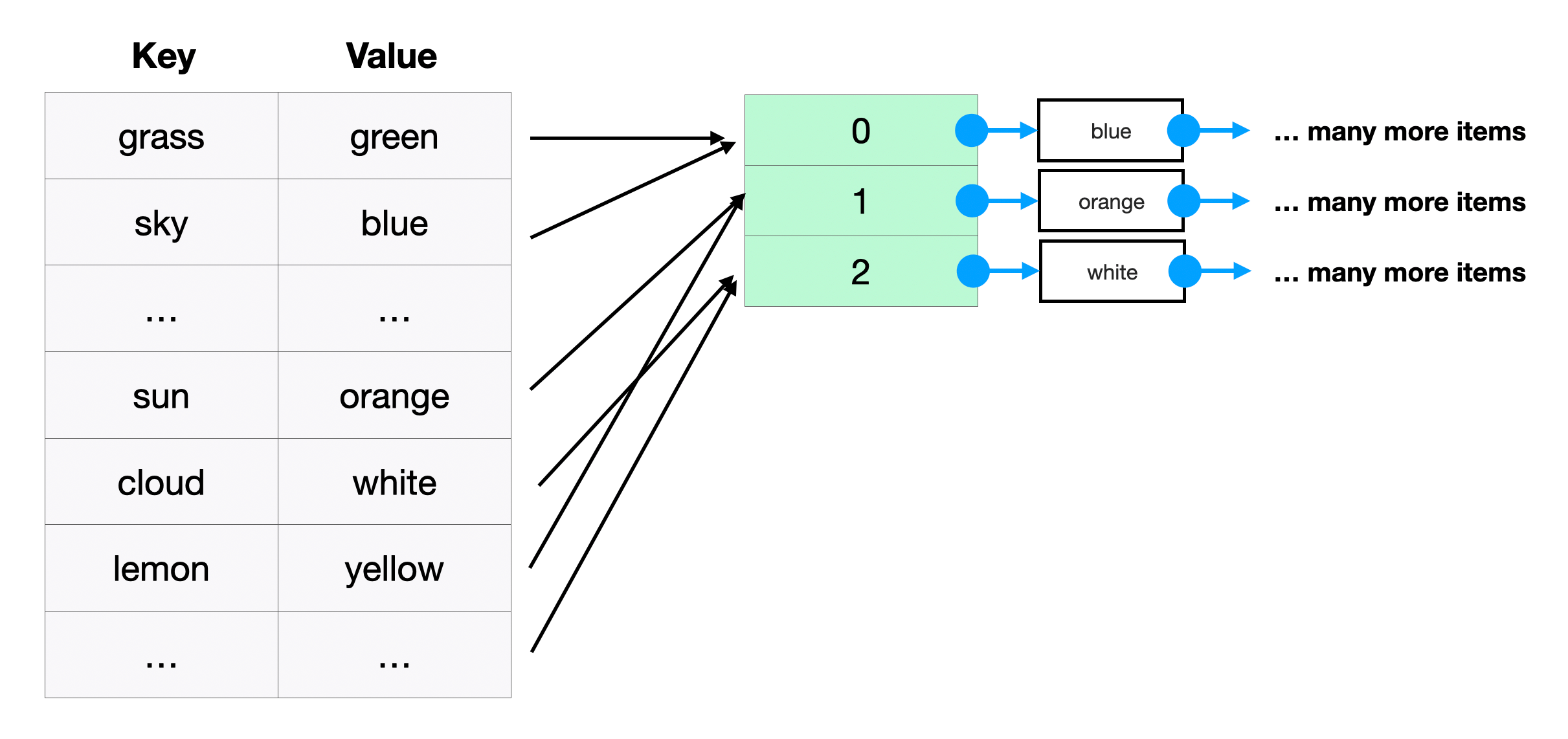Hash Tables | Skilled.dev
Learn

# Hash Tables

Hash tables are one of the most common data structures in coding interviews which makes them essential to master.

The reason hash tables are so important is that they offer constant time O(1) lookup / set / insert / delete. They are frequently used with other data structures to improve the performance of our solution.

In every single interview, you should consider if a hash table could be used to improve your answer.

## Cheat Sheet

Common names: hash, hash table, hash map, dictionary, associative array, map

keyvalue
"grass""green"
"sky""blue"
"sun""orange"
"cloud""white"
"lemon""yellow"
Big O Complexity
lookupO(1)
setO(1)
insertO(1)
deleteO(1)
spaceO(n)
Pros 🔥
• Speed
Fast O(1) operations because keys map directly to a value, allowing us to quickly find/modify/delete the item we want without iterating through the data structure.
• Meaningful and flexible keys
Keys can be declared as strings allowing us to name them in the context of the problem.
• Universal
Key-value storage is easy to send through an API, store in a cache, and access in any language.
Cons 🤕
• No order
Since we store data by key name, we lose meaningful order.
• One-way mapping
We can map keys to a value, but we cannot access the other way around.
• Slow key iteration
If we want to grab all the keys, it requires O(n) time because the only reference to them is stored in the table buckets.

Hash Tables in Interviews:

• Typically you are given an input data structure, and you add a hash table for fast key-value lookups.
• Get O(1) time by trading off to add an additional O(n) space.
• Input data structures will often have order, and the hash table enables constant time lookups on its individual items.

Hash tables in JavaScript:

`` ``

## Hash Tables Explained

Hash tables are very powerful because we can lookup/insert/delete data in constant time O(1) using key-value pairs. Let's take a look at the two important pieces of information from that sentence.

Constant time operations: This means that no matter how much data we are given as the input, a hash table will always be fast.

Store data in key-value pairs: By being able to name the string keys, we give our data meaning. For example, being able to access `inventory.computers` makes our code much more readable.

Hash table keys are similar to array indexes in that they can get constant time operations. However, since arrays only use ordered integers as the indexes, our data can not map to any meaning beyond this. If we stored our `inventory` in an array, reading the item at `inventory` tells a developer nothing, but `inventory.computers` gives direct meaning. String keys provide a powerful abstraction.

The tradeoff for hash tables is that we lose the order of the data. This is why we often pair hash tables with other data structures. The original data structure gives us the input in the desired order, and then we construct the hash table as an additional data structure to be able to look an item up by a meaningful attribute in O(1) time. The trade off is that we add an additional O(n) space complexity by using a hash table.

## How Hash Tables Work Underneath Hood

If this section is confusing, it may become more clear when we implement a hash table.

As we noted, hash tables are very similar to arrays. In fact, hash tables are actually implemented on top of arrays.

Key names have no meaning to a computer, and there is an infinite number of string combinations we could come up with for key names. Computers much more easily handle arrays — their indexes map directly to sequential spots in memory.

So when we initialize a hash table, the programming language actually creates an array underneath the hood. Then when we give the hash table a key, our programming language maps this key to an index in the array.Credit: VisuAlgo

To map the key to an index, our hash table uses something called a hash function. The hash function converts the key input to an integer index in the array. The input to the hash function can be anything (and is therefore infinite), but the array that actually stores the data is a fixed size. This means multiple keys can map to the same index, and we call this a hash collision.

Since multiple keys map to the same index, we have to create a bucket at this index to store the different values it can take. A bucket is typically implemented as a linked list, and each node stores a particular key-value pair. So when we have a hash collision, our hash table will find the bucket, iterate through its linked list, and look for the node that contains the matching key and then return the value.

In the image above, "John Smith" and "Sandra Dee" both hash to 152, so we store both in bucket 152 using a linked list. Notice that it stores both the key and the value. When we look up an item by key, it first returns the hash of the bucket that the key is contained in. Then it iterates through this bucket's linked list to search for the key that we want and returns the associated value.

## Hashing, Hash Functions, and Hash Collisions

Hash functions are a core component of hash tables, but we actually see them all over software development. For example, if you are using GitHub, you may have heard the phrase "commit hash" or noticed that there is a unique string that identifies a commit. What is actually happening is a hash function takes the content of a commit and creates an output hash that uniquely identifies it.

Hash functions take an input of any size and produce a fixed-size output (often an integer represented as a hexadecimal string).

Generate a SHA-256 hash for any input:

Output SHA-256 hash:

There are many ways to implement a hash function, but fundamentally they follow the same format. Popular hashing algorithms include MD5, SHA-1, and SHA-256.

A hash function can also be as simple as taking the UTF-8 code for all the letters in a string and summing them together, which we'll see in a moment.

The input size of a hash function is infinite because we could come up with any string combination to feed it. All hash functions can handle the infinite input, but the output is a fixed/maximum size. Since infinite input values must map to a fixed-size output, we know that entirely different inputs MUST be able to produce identical hashes. This is what's known as a hash collision.

For example, a SHA-256 hash is always 256 bits. This corresponds to 64 hexadecimal characters which is an integer ranging from 0 to 2256. Since the output size is constrained and a fixed length, we know that there are different strings that must produce the same result. This output size is large so a collision is very rare, but the tighter the range of numbers, the higher the likelihood of a collision.

To visualize a hash collision, let's look at a simple hash function that sums the UTF-8 code of the input string characters.

``````s    k    i    l    l    e    d
|    |    |    |    |    |    |
115  107  105  108  108  101  100  =  744

99   111  118  101  114  101  100  =  744
|    |    |    |    |    |    |
c    o    v    e    r    e    d
``````

Totally different words produced the same hash. If we used `skilled` and `covered` as keys for a hash table with that hash function, it would cause a collision, and they would be in the same bucket.

Common characteristics of hash functions include:

• Hash functions are one-way. You can't determine the input based on the output.
• Fixed output size. Hash functions will have a lower and upper bound for values it can produce.
• Produce the same output for a given input. The output of a hash function must be predictable. If you pass the string "skilled.dev" to the SHA-256 algorithm, it will always output `4e6ef22a47c9abbf6f80680f5f814705bf8f793c73eae07e534212f1eb96a4d3`.
• All output values are equally likely. Since the hash function output is limited in size, the distribution of the output must be uniform. This means for a good function, similar words or strings should land in vastly different buckets.
• Work with any input type or scenario. We typically think of passing a hash function either strings or numbers, but it will work with any input, such as an .exe file. At the end of the day, it's all 1's and 0's, and the hash function should know how to handle it.

## Hash Tables O(1) vs. O(n)

In an interview, you can assume a hash table operates in O(1) time. However, to achieve this, our hash table implementation must satisfy some conditions.

1. A good hash function: It assigns keys to buckets evenly (uniformly distributed) and is efficient at calculating the hash.
2. The size of the array is sufficiently large: The hash table's array must be large enough to handle the number of items we store in it without making any bucket too large.

These factors keep collisions low (the number of items sharing a bucket). If the number of collisions is high, it can result in O(n) time, but if the number of collisions is low, it will be O(1).

For example, if we had a bad hash function, it would send too many items to a single bucket instead of distributing them evenly:If the size of the array is too small, the linked lists will grow too large and require ~O(n) time to iterate and find an item.Imagine an extreme scenario where the hash table's array is `length == 1` which means there is one bucket. All `n` key-value pairs would be hashed and stored in this bucket, and so to find any value, we would potentially need to iterate through the entire linked list which requires O(n) time.

To manage this, hash tables resize their storage array based on the number of items that have been added. This ensures there will always be enough buckets.

The hash function also needs to distribute them evenly. If one bucket has a higher probability of getting items, it can grow sufficiently large such that it would effectively take O(n) time to find an item.

In a good hash table implementation, we can assume each bucket has approximately the same number of items. We can also assume that the number of items in a bucket is much smaller than `n`.

Now imagine a hash table with 1,000,000 items that stores them in an array of length 250,000. A good hashing function would mean we store ~4 items per bucket. Even with the collisions, we would only be iterating through 4 nodes to find our key-value pair. Since 4 is significantly lower than our `n = 1000000`, it is constant time.

Collisions are unavoidable, so they just need to be managed effectively which every common programming language does. You can always assume a hash table's operations are performed in O(1) time in your interviews.

Ready for the next step?
Find a Job